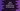# Java program to convert a boolean array to string array## Java program to convert a boolean array to string array:

In this post, we will learn how to convert a Boolean array to a String array in Java. There are basically two methods we can use to convert a Boolean value to String. We will learn both of these methods and how to use them to convert a Boolean array to String array.

### Method 1: By using String.valueOf:

Let’s try with the first method, String.valueOf. This method is defined as like below:

``public static String.valueOf(Boolean value)``

This method takes one Boolean value as the parameter and returns the String value for it.

We can use this method to convert all Boolean values to string in an array. We just need to iterate through the elements of the array.

Below is the complete program:

``````package com.company;

import java.util.Arrays;

public class Main {
public static void main(String[] args) {
boolean[] givenValues = {true, false, true, true, false};
String[] result = new String;

for (int i = 0; i < givenValues.length; i++) {
result[i] = String.valueOf(givenValues[i]);
}

System.out.println(Arrays.toString(result));
}
}``````

Here,

• givenValues is an array of Boolean values.
• result is an array of strings. We have initialized it to size 5.
• The for loop runs through the values of givenValues one by one. For each value we are iterating, we are getting the string value of the Boolean value and adding it to the result array.
• The last line is printing the values of result array.

If you run this program, it will print the below output:

``[true, false, true, true, false]``

## Method 2: By using Boolean.toString:

We have another method in Java to convert a Boolean value to string. It is called toString, defined in Boolean class.

It is defined as like below:

``public static Boolean.toString(boolean value)``

It also returns a string and takes one boolean value as its argument. We can iterate through the array as like the above example and convert each elements to string.

Below is the complete program:

``````package com.company;

import java.util.Arrays;

public class Main {
public static void main(String[] args) {
boolean[] givenValues = {true, false, true, true, false};
String[] result = new String;

for (int i = 0; i < givenValues.length; i++) {
result[i] = Boolean.toString(givenValues[i]);
}

System.out.println(Arrays.toString(result));
}
}``````

If you run it, it print the same output.

``[true, false, true, true, false]``